Courses

# Very Short Answer Type Questions- Lines and Angles Class 9 Notes | EduRev

## Class 9 : Very Short Answer Type Questions- Lines and Angles Class 9 Notes | EduRev

The document Very Short Answer Type Questions- Lines and Angles Class 9 Notes | EduRev is a part of the Class 9 Course Class 9 Mathematics by VP Classes.
All you need of Class 9 at this link: Class 9

Q.1. What is the measure of an angle whose measure is 32° less than its supplement?
Sol.
Let the required angle be x
∴ x = (180°- x) - 32°
⇒ x = 74°

Q.2. If the supplement of an angle is 4 times its complement, find the angle.
Sol.
Let the required angle be x
∴  (180°- x) = 4 (90° - x)
⇒ x = 60°

Q.3. An exterior angle of a Δ is 110° and its two opposite interior angles are equal. What is the measure of each angle?
Sol.
Let each of the interior opposite angle be x
∴ x + x  = 110°
⇒ x = 55°

Q.4. In a rt. ΔABC, ∠A = 90° and AB = AC. What are the values of ∠B and ∠C?
Sol.
∵ AB = AC
⇒ ∠B = ∠C
Also, ∠A = 90°
⇒ ∠B + ∠C = 90°
⇒ ∠B = ∠C = (90o/2) = 45°

Q.5. In the figure, what is the value of x?
Sol.
∵ ℓ || m and p is a transversal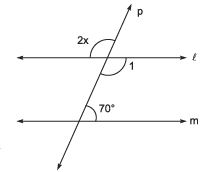∴ ∠1 + 70° = 180°       [co-interior angles]
⇒ ∠1 = 180° - 70° = 110°
Now, 2x = 110°     [vertically opposite angles]
⇒ x =(110°/2) = 55°

Q.6. If two interior angles on the same side of a transversal intersecting two parallel lines are in the ratio 2 : 3 then, what is the smaller angle?
Sol.
ℓ || m and p is the transversal
∴ ‘a’ and ‘b’ are interior angles on the same side of the transversal p.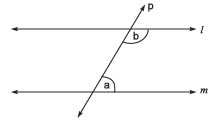Let a = 2x and b = 3x
∴ a + b = 180°
⇒ 2x + 3x = 180°
⇒ 5x = 180°
⇒ x = (180/5)= 36°
∴ smaller angle = 2x = 2 x 36 = 72°

Q.7. In the figure, what is the measure of ∠ ABC?
Sol.
PAQ = ∠BAC     [vertically opp. angles]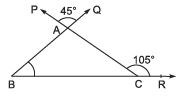Now, ext. ∠ACR= ∠ABC + ∠BAC = 105°
⇒ ∠ABC = 105° - 45° = 60°

Q.8. In the following figure AB || CD. Find the measure of ∠BOC.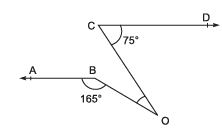Sol. Extending AB to intersect OC, we get the following figure.
ABF is a straight line
∴ ∠OBF = 180° - 165° = 15°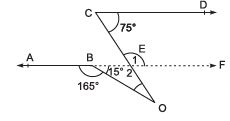AB || CD ⇒ EF || CD
∴ ∠1 + 75° = 180°
⇒ ∠1 = 180° - 75° = 105°
⇒ ∠2 = 105°
Now, in Δ, ∠2 + 15° + ∠BOC = 180°
⇒ 105° + 15° + ∠BOC = 180°
⇒ ∠BOC = 180° - 105° - 15°
= 60°

Offer running on EduRev: Apply code STAYHOME200 to get INR 200 off on our premium plan EduRev Infinity!

125 docs

,

,

,

,

,

,

,

,

,

,

,

,

,

,

,

,

,

,

,

,

,

;